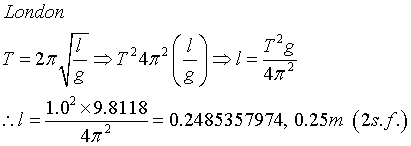# Q6A4-from london to paris with gravity

lemon
1.A pendulum which has a period of 1s in London, where g=9.8118m s-2, is taken to Paris where it is found to lose 20s per day. What is the value of g in Paris?

2. Homework Equations are in the image attached

I like this question but can't figure it out. I have the length of the pendulum correct, I think. I know that there are 86400s in one day; minus 20s for the pendulum period in Paris. Can't figure it out.## Answers and Replies

benhou
What does it mean when it says "lose 20s per day"? Does it mean that in the same amount of periods, it takes 20s less to achieve?

lemon
yeah this is confusing me too. I think it means that in one day the oscillations it usually goes through in England is achieved 20s faster in France. Not sure though.

benhou
It makes the most sense to me if it were so. Now then, find out how much time it loses per period. With the period and the length, you can figure out the gravitational field strength g.

lemon
I just received new:
It means that when the pendulum is taken to Paris, it swings twenty fewer times in 24hrs than when it was in London. (You would say in common parlance that your clock had 'lost time' in such circs, not having ticked enough ticks as it were)

Mindscrape
The wording seems okay to me. There is a pendulum that is calculated to work in london where it's periods coincide with the value of gravity at its location in london, and a certain number of periods elapse to fill a day. Then it's moved to Paris where the value of gravity is slightly different, and the number of periods that fill a day is off now.

So figure out the ratio between the total seconds and relate that back to the different values of gravity. :)

benhou
Looks good. Be careful with the unit of period though. It should be
$$T_{paris}=\frac {86400}{85380}\frac{seconds}{oscillation}$$

lemon
great!
thanks to all y'allwait! how come one is seconds but the other is oscillations?

Last edited: